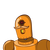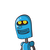# If the l=10cm and b=7cm, the area of the rectangle would be__.

If the l=10cm and b=7cm, the area of the rectangle would be__.

### 2 thoughts on “If the l=10cm and b=7cm, the area of the rectangle would be__.”

1.Step-by-step explanation:

70cm^2

### and volume

2.## Here is the solution

### Given ,

l = 10 cm

b= 7 cm

We know ,

Area of rectangle = l× b

= 10 × 7

= 70 cm

Therefore , area of rectangle is 70 cm